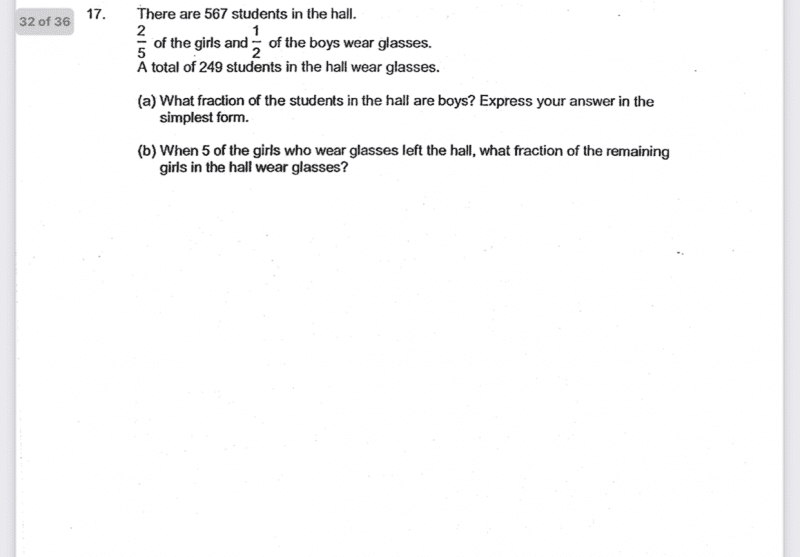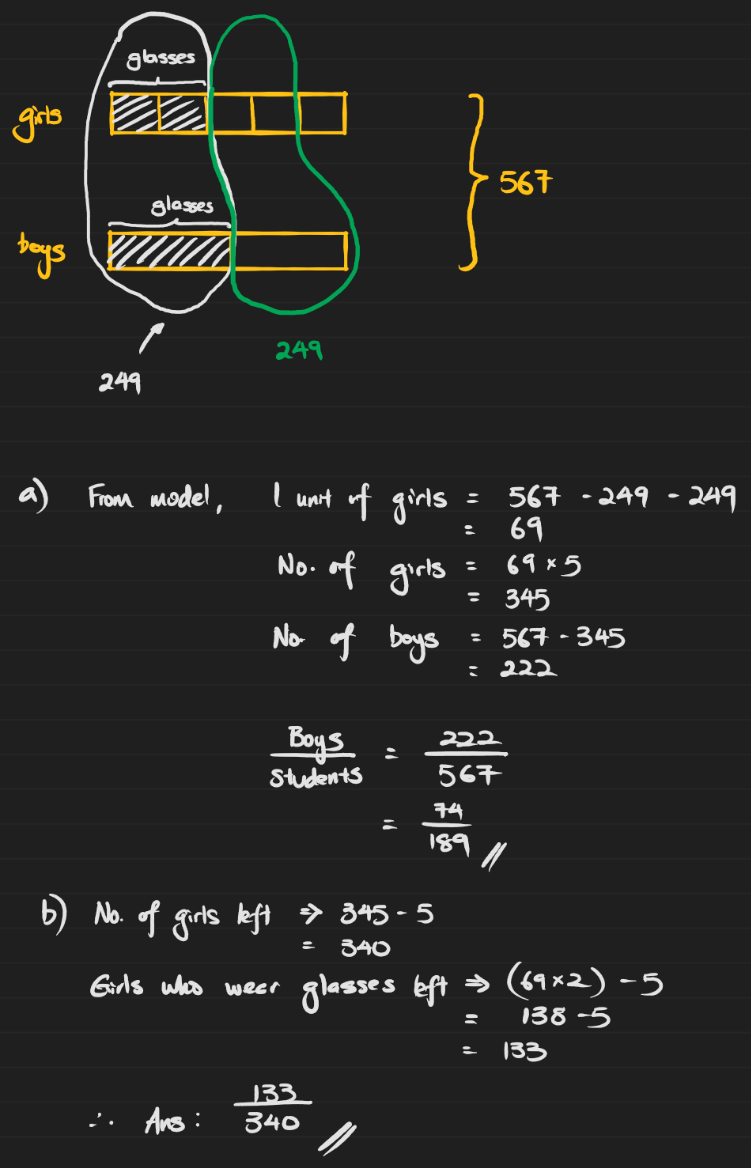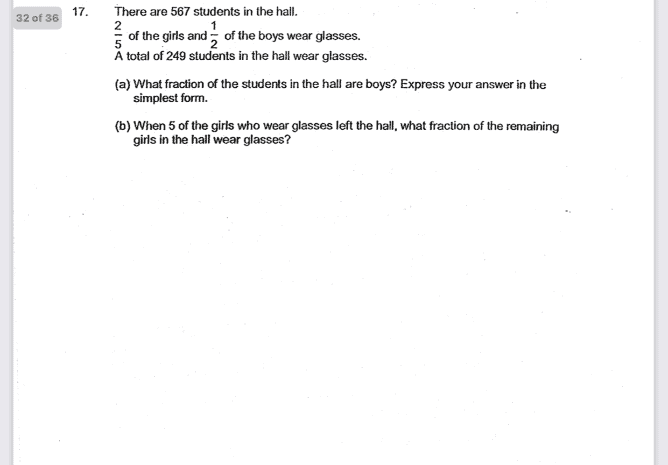# Question0 Replies 2 Likes ✔Accepted Answer

We will set up an equation like this:

2/5X+ 1/2Y= 249

X+Y= 567

x: is the number of female students
Y: is the number of male students

gartic phone

0 Replies 0 LikesUse Elimination Method

Let G represent the girls in the hall.

Let B represent the boys in the hall.

Wear glasses ➜ (2/5)G + (1/2)B = 249 students — Equation (1)

Don’t wear glasses ➜ (3/5)G + (1/2)B = 567 – 249 = 318 students — Equation (2)

Since the fraction of boys who wear glasses and don’t wear glasses are both one half (1/2), subtracting Equation (1) from Equation (2) would eliminate the boys totally (zero), and give a remainder of (1/5)G.

(1/5)G ➜ 318 – 249 = 69 girls

Total girls in hall ➜ 69 x 5 = 345 girls

Total boys in hall ➜ 567 – 345 = 222 boys

Fraction of boys in hall ➜ 222/567 = 74/189 (simplest form) — Part (a) answer

Girls who wear glasses (at first) ➜ 69 x 2 = 138 girls

Girls who wear glasses (new) ➜ 138 – 5 = 133 girls

Total girls in hall (new) ➜ 345 – 5 = 340 girls

Fraction of remaining girls who wear glasses ➜ 133/340 — Part (b) answer

*To learn more problem-solving methods, visit us at Miss J Classroom – Maths is Fun

0 Replies 1 Like